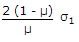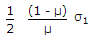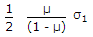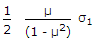# Civil Engineering - UPSC Civil Service Exam Questions - Discussion

32.

A bar of elastic material is subjected to a direct compressive stress σ1 in the longitudinal direction. Suitable lateral compressive stress σ2 is applied along each of the other two lateral directions to limit the net strain in each of the lateral directions to half the magnitude of what it could be under σ1 acting alone. If μ is the Poisson's ratio of the material, then the magnitude of σ2 is

 [A].[B].[C].[D].Explanation:

No answer description available for this question.

 Sanjay Thakur said: (Jan 5, 2017) Can anyone explain this?

 Vai said: (Nov 2, 2017) Consider f1=longitudinal stress. f2 & f3 = lateral stress, m = poisson's ratio. General expression for lateral strain, e2 = f2/E - m*f1/E - m*f3/E. In first case, same stress is applied along each lateral direction. Therefore, f2 = f3. Therefore, e2 = (1-m)*f2/E - m*f1/E. In second case, f1 acting alone. therefore, e2 = - m*f1/E. Condition given is, lateral strain in first case = half of lateral strain in second case. (1-m)*f2/E - m*f1/E = - 0.5*m*f1/E. (1-m)*f2/E = 0.5*m*f1/E. f2 = 0.5* [m/(1-m)] *f1. Hence C is correct option.